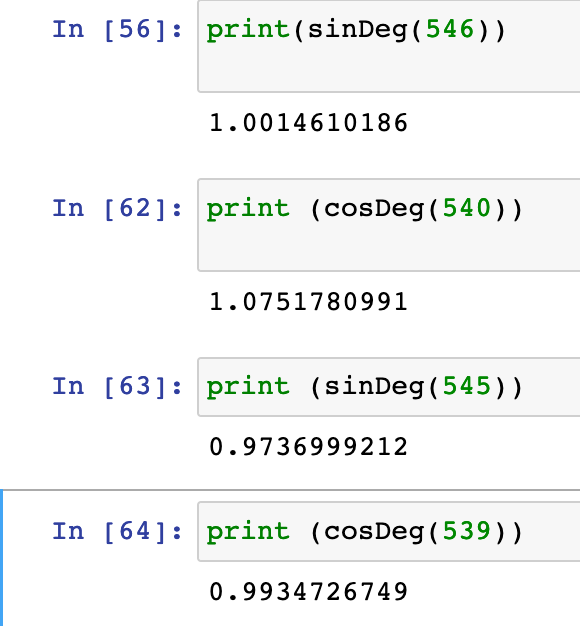Instantly share code, notes, and snippets.

# xtrp/sin_cos_functions.py

Created November 26, 2020 20:21
Star You must be signed in to star a gist
Maclaurin/Taylor Series Calculation of Sin and Cos in Python
This file contains bidirectional Unicode text that may be interpreted or compiled differently than what appears below. To review, open the file in an editor that reveals hidden Unicode characters. Learn more about bidirectional Unicode characters
 from math import pi # round a number (x) to nearest 10 digits def rounded(x): return round(x, 10) # get the factorial of a number (x) # factorial(x) is the product of every number from 1 to N inclusive def factorial(x): n = 1; # n is the result # multiply n by every number from 1 to x inclusive for i in range(2, x + 1): n *= i return n """ get the result of the cos and sin formulas where the functions are sin(x radians) or cos(x radians), n is the start value (n = x for sin, n = 1 for cos), and i_start is the exponent and factorial base in the first term """ def computeSeries(x, n, i_start): iterations = 20 # iterations is twice the amount of terms to use multiplier = 1 for i in range(i_start, i_start + iterations, 2): # i increases by 2 each term multiplier *= -1 # alternates between addition and subtraction each term next_term = (x**i) / factorial(i) # each term is (x^i) / i! n += multiplier * next_term # add or subtract from final result return n # get sin of x radians def sin(x): return rounded(computeSeries(x, x, 3)) # get cos of x radians def cos(x): return rounded(computeSeries(x, 1, 2)) # get sin of x degrees def sinDeg(x): return sin(x * pi / 180) # get cos of x degrees def cosDeg(x): return cos(x * pi / 180) # test the functions print(sin(pi / 6)); # 0.5 print(sinDeg(45)); # 0.7071 print(sinDeg(52)); # 0.78801 print(cos(pi / 3)); # 0.5 print(cosDeg(45)); # 0.7071 print(cosDeg(52)); # 0.615661

### nagarajasr commented Jan 4, 2021 • edited

the code seems to break at certain values of x. sinDeg(x) gives a result > 1 at x=546 similarly cosDeg(x) gives a result > 1 at x=540
tested in Python 3.9.1
jupyter core : 4.7.0
jupyter-notebook : 6.1.6